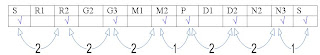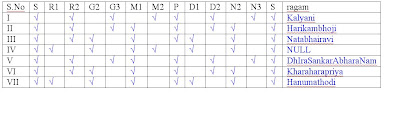## Monday, August 20, 2007

In his post on Vaidehi Raman, PRC wrote in one of his comments---

I realized there is a way of communicating sruthi bhedam to engineers.
Assume sa = 1, ri=2, ga = 3, ma=4, pa=5, dha=6, ni=7 (assuming all swaras are in kalyani)

If f(1)=Kalyani then
f(2) = hari kambhoji
f(3) = nata bhairavi
f(4) = is a null set
f(5) = shankaraabharanam
f(6) = kharaharapriya
f(7) = hanumathodi

if it is of any help!

This post elaborates more on the procedure and hopefully makes it simpler.

To me, this seems like a three step procedure to find out which ragas can be obtained by applying shrutibhedam on a given raga:

Consider Kalyani as above (since we already knowthe final answers... so no doubts there!)

Kalyani: S R2 G3 M2 P D2 N3 S

STEP-I:
Drawing the swara line, analogous to the number line of the Mathematics, we can get the distances between two successive swaras:So Kalyani can be represented as 2 2 2 1 2 2 1.

STEP-II:
Now, keep applying left shift to this sequence to make each successive note as the base note (Shadjamam). We get the following series of sequences:In the above series, sequence no. II represents the scale when Rishabham of Kalyani is made as the base note, i.e., Shadjamam; III represents the scale when GAndhAram of Kalyani is made Shadjamam, etc.

STEP-III:
Now let us see what raga each of the above sequences turns out to be—The last column lists the name of the scale hence obtained as a result of transformation.

So there we are!

Similarly, shruti-bhedam can be performed on janya ragas as well:

Consider Mohanam: S R2 G3 P D2 S. It can be encoded as 2 2 3 2 3 from the swara line in step- I. Now, we can apply the same procedure as above to Mohanam and see what ragas we can get out of it!

There are similar methods given on various websites related to Carnatic and this post is based on what I have learned from those websites.

So it turns out that this shrutibhedam concept is not too difficult atleast to understand... to sing or to play using Shrutibhedam is another matter though...

Kedar.